# 基于MapReduce的语义网空间数据关联A Map-Reduce-Based Parallel Approach for Geospatial Data Interlinking in a Semantic Web

DOI: 10.12677/GST.2019.72014, PDF, HTML, XML, 下载: 651  浏览: 1,017

Abstract: The Web of Data represents an intermediate step towards the Semantic Web. Constructing links among different Resource Description Framework (RDF) datasets is a key issue in the Web of Data. An identity link aims to match entities from different datasets and is an important type of RDF link. There are many approaches to constructing identity links between geospatial entities. This paper adopts the Hausdorff distance to compute the location and shape similarity between two entities. Because the computation of the Hausdorff distance is complex and geospatial data intrinsically large, the entire matching process is very time consuming. This paper proposes a Map-Reduce-based framework to parallelize the similarity computation, significantly reducing the runtime. This approach was verified to be effective in an experiment using data from Nomenclature of Territorial Units for Statistics (NUTS) and Database of Global Administrative Areas (GADM). The matching precision was high, and with the utilization of the proposed parallel framework, the runtime was reduced to only approximately 3 h on 8 nodes; in contrast, when run on 1 node, the runtime exceeded one day.

1. 引言

2. 地理空间实体的同质关联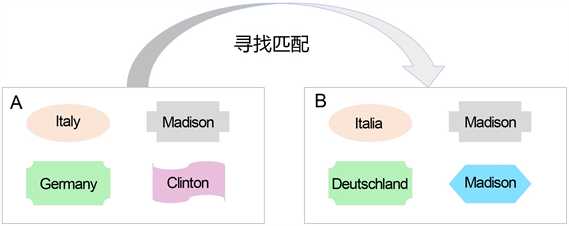Figure 1. Finding matches between geospatial datasets A and B

3. 方法

3.1. Hausdorff相似性度量

Hausdorff距离隐含地计算了实体之间的位置和形状相似度，非常适合用于计算地理空间实体的空间相似度。

$H\left(A,B\right)=\mathrm{max}\left(h\left(A,B\right),h\left(B,A\right)\right)$ (1)

$h\left(A,B\right)={\mathrm{max}}_{a\in A}{\mathrm{min}}_{b\in B}‖a-b‖$ (2)

 (3)

$sim\left(A,B\right)=1-\frac{H\left(A,B\right)}{{L}_{D}}$ (4)

3.2. 基于MapReduce的并行匹配框架

MapReduce是大型数据集并行处理的编程模型  。模型的所有输入和输出都是键/值对的形式。该模型有两个主要函数：Map和Reduce。Map接收一个输入键值对，以用户自定义的方式处理输入数据并生成中间键/值对。然后，MapReduce库将这些中间键/值对分成许多组，每组含有相同的键，并将它们传递给Reduce。每个Reduce都会接收一个键和该键的一组值，以用户自定义的方式处理它们并生成新的键/值对作为输出。Table 1. Hausdorff similarity-matching algorithm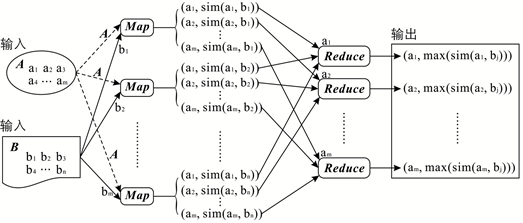Figure 2. Map-Reduce workflow. Dataset B is split using Map-reduce, whereas dataset A is shared

4. 实验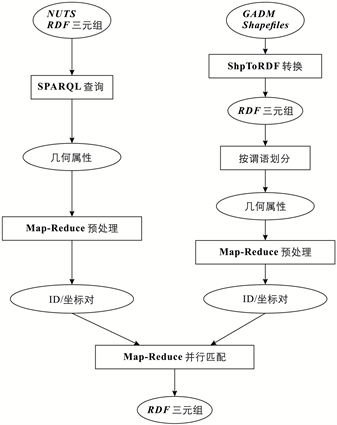Figure 3. Experiment workflow

4.1. 数据预处理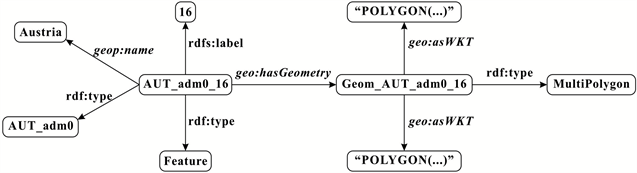(a)(b)

Figure 4. The data for Austria. (a) Austrian data shown in the RDF graph. (b) Austrian data shown in N-TRIPLES format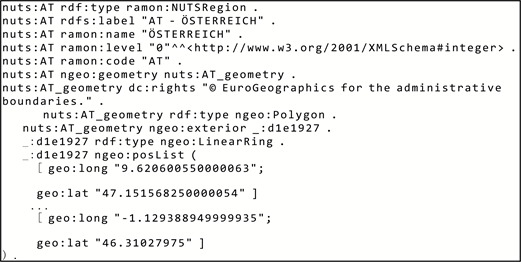Figure 5. A portion of the Austrian data in NUTS

4.2. 实体匹配

4.3. 匹配结果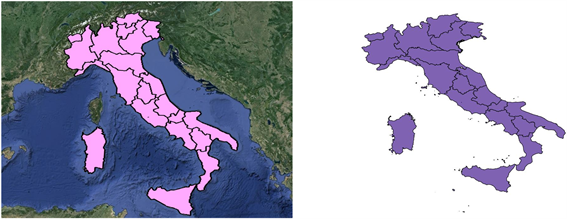Figure 6. Illustration of the data for Italy: Data from NUTS (left) and data from GADM (right)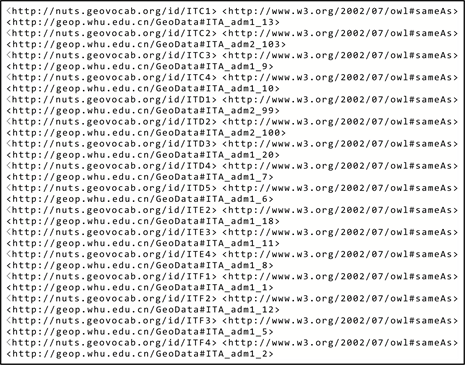Figure 7. Published triples of Italy

4.4. 运行时间和可扩展性Table 3. Speedup as the number of nodes increases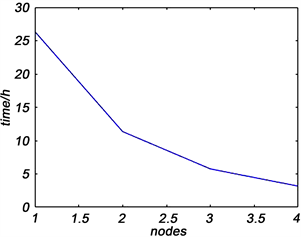Figure 8. Runtime as a function of the number of nodes

5. 讨论

6. 结论